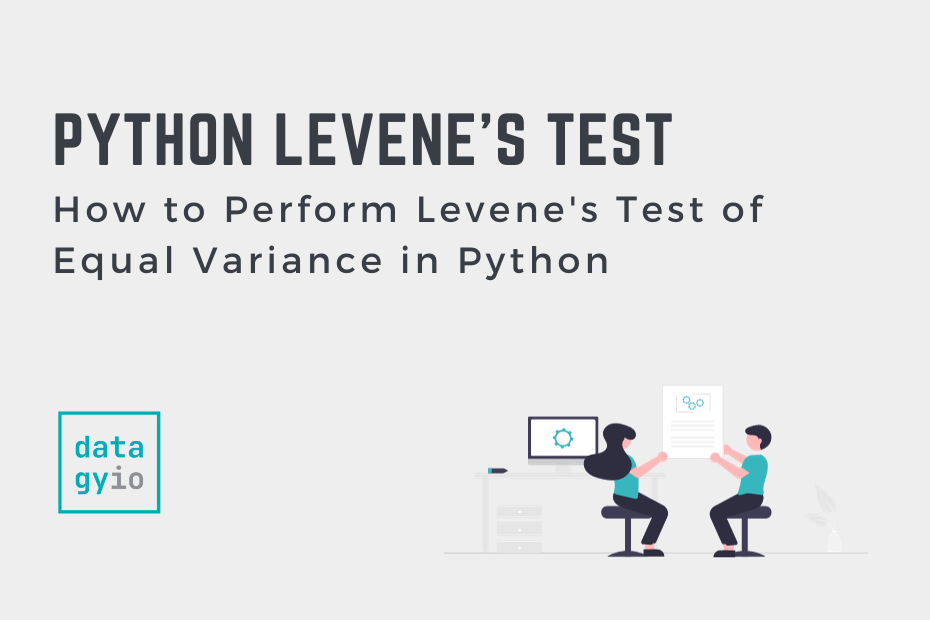# Blog## How to Perform Levene’s Test of Equal Variance in Python

Levene’s test is an important statistical test used to assess the equality of variance between different samples. In this tutorial, you’ll learn how to understand and compute Levene’s test of equal variance in Python, using the important scipy package. Since… Read More »How to Perform Levene’s Test of Equal Variance in Python## How to Calculate R-Squared in Python (SkLearn and SciPy)

Welcome to our exploration of R-squared (R2), a powerful metric in statistics that assesses the goodness of fit in regression models. R2 represents the proportion of the variance in the dependent variable that is predictable from the independent variable(s). In… Read More »How to Calculate R-Squared in Python (SkLearn and SciPy)## How to Create Tables Easily in Python with Tabulate

Python makes it simple to display data in table format using the tabulate() function from the tabulate library. This can be a helpful way of understanding your data, rather than simply printing lists, dictionaries, or other Python objects. In this… Read More »How to Create Tables Easily in Python with Tabulate## Calculate the Coefficient of Variation in Python (SciPy, NumPy, Pandas)

The coefficient of variation, or CV, allows you to measure how spread out values in a dataset are, relative to their mean. In this tutorial, you’ll learn how to interpret the coefficient of variation and how to calculate it in… Read More »Calculate the Coefficient of Variation in Python (SciPy, NumPy, Pandas)

## How to Use Python to Test for Normality

In this tutorial, you’ll learn how to check the assumption that data in a dataset are distributed normally, meaning that they follow a Gaussian distribution. This is an incredibly important topic to understand, given how many statistical tests make the… Read More »How to Use Python to Test for Normality## Tanh Activation Function for Deep Learning: A Complete Guide

In this comprehensive guide, you’ll explore the Tanh activation function in the realm of deep learning. Activation functions are one of the essential building blocks in deep learning that breathe life into artificial neural networks. The Tanh activation function is… Read More »Tanh Activation Function for Deep Learning: A Complete Guide## Softmax Activation Function for Deep Learning: A Complete Guide

In this comprehensive guide, you’ll explore the softmax activation function in the realm of deep learning. Activation functions are one of the essential building blocks in deep learning that breathe life into artificial neural networks. The softmax activation function is… Read More »Softmax Activation Function for Deep Learning: A Complete Guide## ReLU Activation Function for Deep Learning: A Complete Guide to the Rectified Linear Unit

In the world of deep learning, activations breathe the life into neural networks by introducing non-linearity, enabling them to learn complex patterns. The Rectified Linear Unit (ReLU) function is a cornerstone activation function, enabling simple, neural efficiency for reducing the… Read More »ReLU Activation Function for Deep Learning: A Complete Guide to the Rectified Linear Unit## Mean Absolute Error (MAE) Loss Function in PyTorch

In this tutorial, you’ll learn about the Mean Absolute Error (MAE) or L1 Loss Function in PyTorch for developing your deep-learning models. The MAE loss function is an important criterion for evaluating regression models in PyTorch. This tutorial provides a… Read More »Mean Absolute Error (MAE) Loss Function in PyTorch## How to Normalize NumPy Arrays (Min-Max Scaling, Z-Score, L2)

In this tutorial, you’ll learn how normalize NumPy arrays, including multi-dimensional arrays. Normalization is an important skill for any data analyst or data scientist. Normalization refers to the process of scaling data within a specific range or distribution to make… Read More »How to Normalize NumPy Arrays (Min-Max Scaling, Z-Score, L2)## PyTorch Loss Functions: The Complete Guide

In this guide, you will learn all you need to know about PyTorch loss functions. Loss functions give your model the ability to learn, by determining where mistakes need to be corrected. In technical terms, machine learning models are optimization… Read More »PyTorch Loss Functions: The Complete Guide## Pandas GroupBy Multiple Columns Explained with Examples

The Pandas groupby method is a powerful tool that allows you to aggregate data using a simple syntax, while abstracting away complex calculations. One of the strongest benefits of the groupby method is the ability to group by multiple columns,… Read More »Pandas GroupBy Multiple Columns Explained with Examples